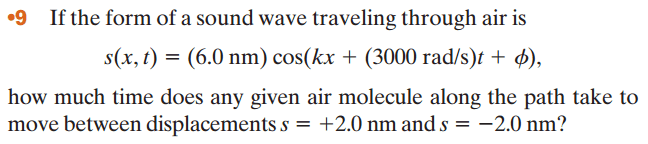# Time between sound wave displacements

## Homework Statement## The Attempt at a Solution

So I set up:

2.0 nm = (6.0 nm)cos(kx + (3000 rad/s)t1 + phi)
-2.0 nm = (6.0 nm)cos(kx + (3000 rad/s)t2 + phi)

My professor said if there's a phi you can usually ignore it so I'm just going to remove them:

2.0 nm = (6.0 nm) * cos(kx + (3000 rad/s)t1)
-2.0 nm = (6.0 nm) * cos(kx + (3000 rad/s)t2)

Simplify them:

1/3 nm = cos(kx + (3000 rad/s)t1)
-1/3 nm = cos(kx + (3000 rad/s)t2)

I need to solve for each time, but we have 3 unknowns and only two equations so I'm not sure what else to do from here.

## Answers and Replies

One thing you can do is set $t_0$ to zero. Then you can set $x$ to zero, too.

One thing you can do is set $t_0$ to zero. Then you can set $x$ to zero, too.

But then we get

s = (6.0 nm) * cos(k*0 + (3000 rad/s)*0)
s = 6.0 nm * 1
s = 6.0 nm

I'm not sure how this helps

But then we get

s = (6.0 nm) * cos(k*0 + (3000 rad/s)*0)
You left out the phase constant.

Oh...I'm not sure when to include the phase constant or not because sometimes it seems negligible and when we are doing problems the professor just leaves it out. But doesn't that add another variable anyway?

s = (6.0 nm) * cos(phi)

Well, if you want to omit $\phi$, then stick $x$ back in. But then you'll have to worry about $k$, too.

Well, if you want to omit $\phi$, then stick $x$ back in. But then you'll have to worry about $k$, too.

I don't understand this relationship. Why does x and k re-appear if phi disappears?

If you set your frame of reference so that x = 0 and t1 = 0, then you have to specify the starting phase through phi in order to get the required initial displacement 2.0 nm.

One thing you can do is set $t_0$ to zero. Then you can set $x$ to zero, too.
I meant $t_1$.

how can I calculate the value of phi to accomodate for the different starting point?

The starting phase must be such that the 6.0 sin (phase) = 2.0. This follows directly form the description of the problem.